## Example Questions

### Example Question #196 : Plane Geometry

What is the area of an isosceles right triangle with a hypotenuse of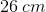?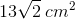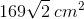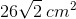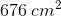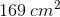Explanation:

Now, this is really your standard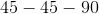triangle. Since it is a right triangle, you know that you have at least one-degree angle. The other two angles must each bedegrees, because the triangle is isosceles.

Based on the description of your triangle, you can draw the following figure: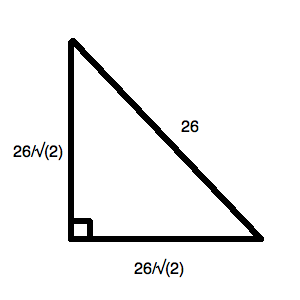This is derived from your reference triangle for thetriangle: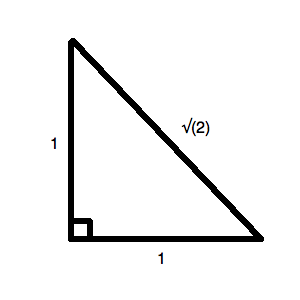For our triangle, we could call one of the legs. We know, then: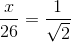Thus,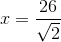.

The area of your triangle is: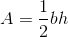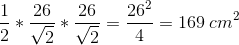### Example Question #197 : Plane Geometry

What is the area of an isosceles right triangle with a hypotenuse of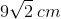?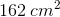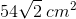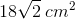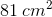Explanation:

Now, this is really your standardtriangle. Since it is a right triangle, you know that you have at least one-degree angle. The other two angles must each bedegrees because the triangle is isosceles.

Based on the description of your triangle, you can draw the following figure: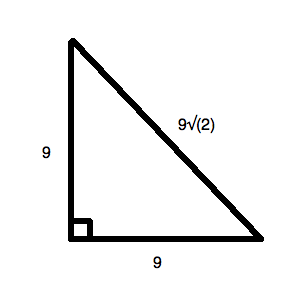This is derived from your reference triangle for thetriangle:For our triangle, we could call one of the legs. We know, then: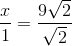Thus,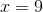.

The area of your triangle is: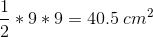### Example Question #198 : Plane Geometry

What is the area of an isosceles right triangle with a hypotenuse of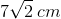?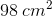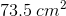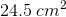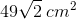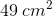Explanation:

Now, this is really your standardtriangle. Since it is a right triangle, you know that you have at least one-degree angle. The other two angles must each bedegrees because the triangle is isosceles.

Based on the description of your triangle, you can draw the following figure: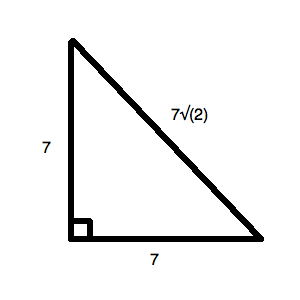This is derived from your reference triangle for thetriangle:For our triangle, we could call one of the legs. We know, then: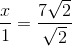Thus,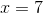.

The area of your triangle is: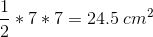### Example Question #199 : Plane Geometry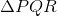is a right isosceles triangle with hypotenuse. What is the area of?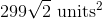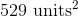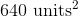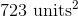Explanation:

Right isosceles triangles (also called "45-45-90 right triangles") are special shapes. In a plane, they are exactly half of a square, and their sides can therefore be expressed as a ratio equal to the sides of a square and the square's diagonal: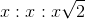, where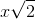is the hypotenuse.

In this case,maps to, so to find the length of a side (so we can use the triangle area formula), just divide the hypotenuse by: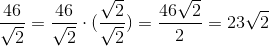So, each side of the triangle is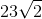long. Now, just follow your formula for area of a triangle: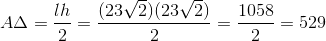Thus, the triangle has an area of.